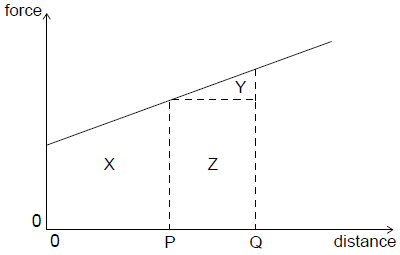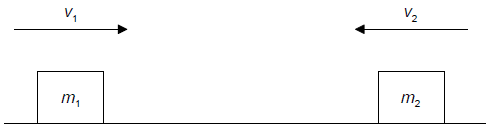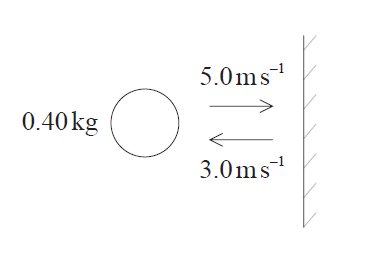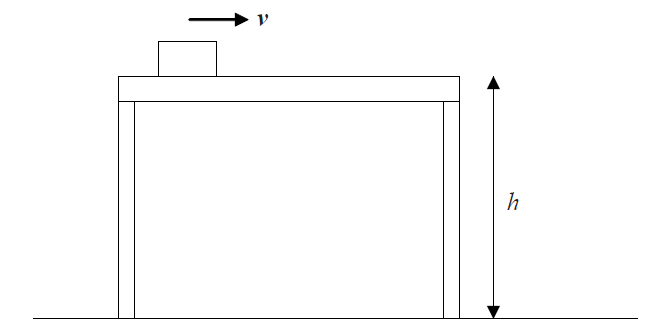1. Two objects undergo an inelastic collision. Which of the following is correct in respect of both the conservation of momentum and the conservation of total energy of the system?2. A gas atom strikes a wall with speed v at an angle θ to the normal to the wall. The atom rebounds at the same speed v and angle θ .Which of the following gives the magnitude of the momentum change of the gas atom?

3. The initial kinetic energy of a block moving on a horizontal floor is 48 J. A constant frictional force acts on the block bringing it to rest over a distance of 2 m. What is the frictional force on the block?
4. Objects A and B collide together. They end up joined together and stationary. During the collision, a force + F is exerted on object A by object B . According to Newton’s third law, there will also be a force of
5. An object falls from rest from a height h close to the surface of the Moon. The Moon has no atmosphere.

When the object has fallen to height  h/4 above the surface, what is6. A car travelling at a constant velocity covers a distance of 100 m in 5.0 s. The thrust of the engine is 1.5 kN. What is the power of the car?
7. An object is at rest at time t = 0 . The variation with t of the acceleration a of the object is shown from t = 0 to t = 20 s .What is the speed of the object when t=15 s?

8. A boy runs along a straight horizontal track. The graph shows how his speed v varies with time t.After 15 s the boy has run 50 m. What is his instantaneous speed and his average speed when = 15 s?9. A graph shows the variation of force acting on an object moving in a straight line with distance moved by the object. Which area represents the work done on the object during its motion from P to Q?10. Two objects

m1 and m2 approach each other along a straight line with speeds v1 and v2 as shown. The objects collide and stick together.What is the total change of linear momentum of the objects as a result of the collision?

11. Two balls of different mass are dropped from the top of a tall building one after the other. The distance between the balls
12. Which of the following is a condition for an object to be in translational equilibrium?
13. A projectile is fired horizontally from the top of a cliff. The projectile hits the ground 4 s later at a distance of 2 km from the base of the cliff. What is the height of the cliff?
14. The momentum of a particle stays constant provided that
15. The diagram shows a girl attempting (but failing) to lift a heavy suitcase of weight W . The magnitude of the vertical upwards pull of the girl on the suitcase is P and the magnitude of the vertical reaction of the floor on the suitcase is R .Which equation correctly relates W, P and R?

16. A net force acts on a body. Which characteristic of the body will definitely change?
17. A stone is thrown vertically upwards from the surface of Earth. Which of the following quantities will not become zero while the stone is in the air?
18. A railway engine of mass m moves along a horizontal track with uniform speed v . The total resistive force acting on the engine is F .Which of the following is the power of the engine?

19. A ball of mass 0.40 kg travels horizontally and strikes a vertical wall with a speed of 5.0

ms–1. It rebounds horizontally with a speed of 3.0 ms–1. The ball is in contact with the wall for a time of 0.20 s.What is the average magnitude of the force exerted by the ball on the wall?

20. An object is projected vertically upwards at time t = 0. Air resistance is negligible. The object passes the same point above its starting position at times 2 s and 8 s.

If g = 10 m s–2, what is the initial speed of the object?

21. Which of the following is proportional to the net external force acting on a body?
22. Which statement applies to an object in translational equilibrium?
23. A lift (elevator) is operated by an electric motor. It moves between the

10th floor and the 2nd floor at a constant speed. One main energy transformation during this journey is

24. Child X throws a ball to child Y. The system consists of the ball, the children and the Earth. What is true for the system when the ball has been caught by Y?25. The graph shows how the displacement d of an object varies with time t . The tangent to the curve at time

t1 is also shown.Which of the following gives the speed of the object at point P?

26. An object, initially at rest, travels a distance d in a time t at a constant acceleration. What is the time taken for the object to travel 16d from rest at the same acceleration?
27. Which of the following is an elastic collision?
28. An egg dropped on the floor is likely to break. However, when it is wrapped in a cloth it is less likely to break. This is because the cloth
29. The distance travelled by the object during the first 4.0 seconds is
30. An object of mass

1 has a kinetic energy E1. Another object has a mass m2 and kinetic energy E2. The objects have the same momentum. What is the ratio E_1/E_2?

31. The graph shows the variation with time t of the force F acting on an object of mass 15 000 kg.

The object is at rest at = 0.What is the speed of the object when = 30 s?

32. A constant force of 12 N is applied for 3.0 s to a body initially at rest. The final velocity of the body is.

6.0m s–1. What is the mass of the body?

33. A block of mass m is moving at constant velocity v along a frictionless surface that is height h above the ground.Which expression gives the work necessary to maintain the constant velocity?

34. A vehicle is driven up a hill at constant speed. Which of the following best describes the energy changes involved?
35. Each side of a metal cube is measured to be 2.0 cm ± 0.20 cm. What is the absolute uncertainty in the calculated volume of the cube?
36. A rubber ball, travelling in a horizontal direction, strikes a vertical wall. It rebounds at right angles to the wall. The graph below illustrates the variation of the ball’s momentum p with time t when the ball is in contact with the wall.Which of the following statements is true?

37. Two balls X and Y with the same diameter are fired horizontally with the same initial momentum from the same height above the ground. The mass of X is greater than the mass of Y. Air resistance is negligible.

What is correct about the horizontal distances travelled by X and Y and the times taken by X and Y to reach the ground?38. The time taken for a stone dropped from rest to fall vertically through 16 m is 2.0 s. Based on these measurements, what is the best estimate for the acceleration of free fall?
39. Two identical spheres, each of mass m and speed v, travel towards each other on a frictionless surface in a vacuum.The spheres undergo a head-on elastic collision.

Which statement correctly describes the spheres after the collision?

40. A force which increases uniformly from 0 to a maximum value of F is applied to an object. The object does not move until the force exceeds 0.5F. As the force increases from 0.5F to F the object moves a distance x in the direction of the force. What is the work done by this force?
41. A student makes three statements about situations in which no work is done on an object.

I. The object is moving with uniform circular motion.
II. A force is applied to the object in the direction of its velocity.
III. A force is applied to the object in a direction opposite to its motion.

Which of the above statements is/are correct?

42. Which of the following is the condition for a body to be in translational equilibrium?
43. An ice-hockey puck is slid along ice in a straight line. The puck travels at a steady speed of 20

ms–1 and experiences no frictional force. How far does the puck travel in 2.5 s?

44. An object is released from rest in the gravitational field of the Earth. Air resistance is negligible. How far does the object move during the fourth second of its motion?
45. A ball of mass m travels horizontally with speed v before colliding with a vertical wall. The ball rebounds at speed v in a direction opposite to its initial direction. What is the magnitude of the change in momentum of the ball?